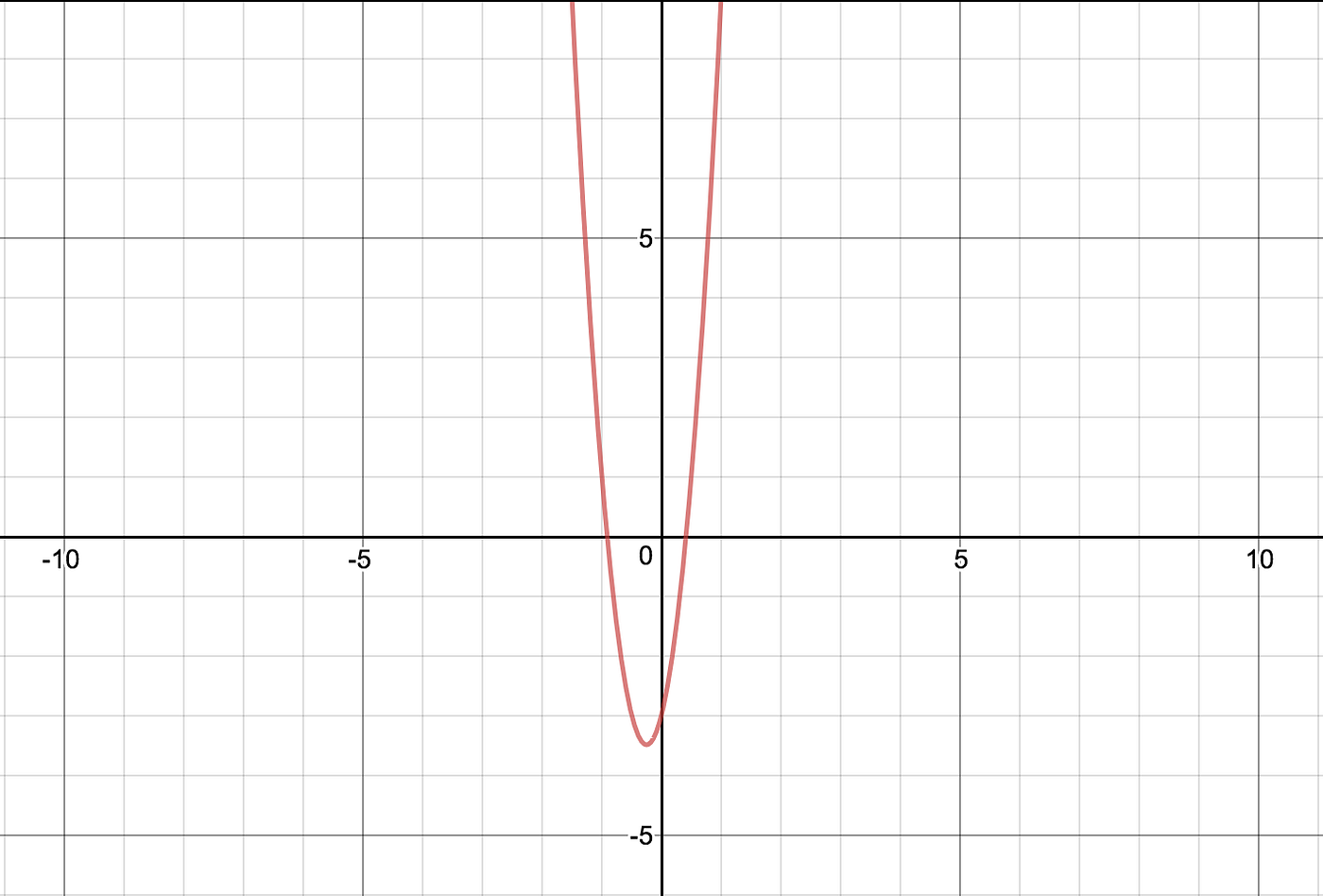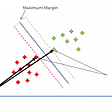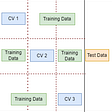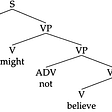# Learning a quadratic equation with PyTorch: Intro to PyTorch

I tried out PyTorch after hearing all hype surrounding the framework and I have never looked back!
Coming from a Tensorflow background, the simplicity of PyTorch was so very alien and surely it couldn’t be this easy. I assure you though, it is!

In this post we will first introduce PyTorch, keywords and concepts, and build a simple feedforward neural network that will learn the underlying function of the given quadratic equation below:f(x) = y = 8x² + 4x — 3 : desmos.com

This post aims to get our feet wet in PyTorch. To install PyTorch follow the instructions on the website pytorch.org.

PyTorch is awesome!
- Abraham Lincoln

# Introduction to PyTorch:

The PyTorch website describes the framework as “a deep learning framework that puts Python first.”

To me, this is a simple yet powerful way to describe the framework, in the sense that, unlike some of the other frameworks out there, I am able to take my current (imperative) python skills and mindset and simply apply it in the context of deep learning.

At first, this line just felt like a cool catch phrase, until we got to debugging — debugging deep neural networks has never been easier! You get the error, AND the stacktrace! Allowing you to find out exactly where the issues lie. As an added bonus, you can set up print statements to quickly see the shape of your tensors or anything else you want to inspect (or change?) as the model is running.

If you have any experience with the VERY popular NumPy library, PyTorch is a tensor library that works like NumPy with extras like GPU support for faster training times, automatic differentiation of gradients, optimizer functions, and so much more to make research and developing deep learning models possible and easier.

# Key words & concepts

Deep learning is a machine learning subfield that is inspired by the working of the brain, particularly the visual cortex. We take the idea that the brain consists of layers of neurons that fire depending on the input they receive from the previous neuron, and so on and so forth.

A Tensor is a multi-dimensional matrix containing elements of a single data type. i.e: [[2, 4, 5], [5, 9, 2]] is a 2 x 3 Tensor.

Variables are simply thin wrappers around Tensors that record the operations that have happened to the Tensor. They are part of PyTorch’s own automatic differentiation package, `torch.autograd.Variable` , and are also responsible for holding the gradient with respect to the tensor they are wrapping.

A Loss function, also known as a cost function, is a function that takes the expected values and the predicted values from our neural network, and calculates how wrong our prediction is. The “wrongness” value is known as the loss or cost and is what we are constantly trying to minimize as we train our model. Typically, the lower this value is, the better our model performs (more on overfitting in another post).

An Optimizer is a function that updates the weights across of the neurons in the model to help the model predict a better output on the next try. PyTorch implements the `torch.optim` package that contains many of the popular optimization algorithms like SGD, Adam, RMSProp and MANY MORE.

# Let’s code!

I will walk through the code, explaining what every line does. The full code can be found at the end of the post.

First, we import the modules that we need:

`# import the required packagesimport torchfrom torch import Tensorfrom torch.nn import Linear, MSELoss, functional as Ffrom torch.optim import SGD, Adam, RMSpropfrom torch.autograd import Variableimport numpy as np`

Above we have imported the torch module (PyTorch), and imported other packages like loss functions and optimizers from torch to make typing easier later on. We have also imported our trusted numpy package.

From torch we have imported `Tensor`, a handy wrapper to our matrices.

From `torch.nn` we import `Linear` which applies the linear transformation: `y=Ax + b` — where A is the gradient and b is the bias. We also import MSELoss which is a loss function that calculates the mean squared error from the difference between the prediction and the expected value. Lastly, we import the module `functional` and alias it as F. This is simply a functional interface that lets us do some extra customization, and also saves on typing as you will see in a bit.

From `torch.optim` we are importing our optimizer algorithms, these are already implemented out of the box and its just plug and play! We import the fundamental Stochastic Gradient Descent, and my two go to algorithms Adam and RMSProp, to play with and see if there will be any improvement.

Last but not least, we import the Variable wrapper from `torch.optim`. This is essential for allowing our weights to be computed and adjusted for the model to perform better.

## Create our data generation utility function:

Since we are just practicing on a quadratic function, we can pick any function and try to train our model to understand the underlying equation. For this example I chose the modest function: `f(x) = y = 8x² + 4x — 3`

`# define our data generation functiondef data_generator(data_size=50):    # f(x) = y = 8x^2 + 4x - 3    inputs = []    labels = []        # loop data_size times to generate the data    for ix in range(data_size):                # generate a random number between 0 and 1000        x = np.random.randint(1000) / 1000                # calculate the y value using the function 8x^2 + 4x - 3        y = 8*(x*x) + (4*x) - 3                # append the values to our input and labels lists        inputs.append([x])        labels.append([y])            return inputs, labels`

The above function is just a crude way for us two generate 2 lists, one containing the X value and the other containing the Y value.

The function takes a data size (default = 50), creates a peudo-random number between 0 and 1000 (X) and passes it through our quadratic `y = 8x² + 4x — 3` to generate our output (y). Do this 50 times, assuming 50 is the data_size, and return two lists of data.

## Define our neural network model:

PyTorch makes it super easy to define your model in a pythonic and familiar way.

Note: The sequential method is even easier than the method I have chosen!

`# define the model class Net(torch.nn.Module):    def __init__(self):        super(Net, self).__init__()        self.fc1 = Linear(1, 6)        self.fc2 = Linear(6, 6)        self.fc3 = Linear(6, 1)            def forward(self, x):        x = F.dropout(F.relu(self.fc1(x)), p=0.5)        x = F.relu(self.fc2(x))        x = self.fc3(x)        return x    model = Net()# define the loss functioncritereon = MSELoss()# define the optimizeroptimizer = SGD(model.parameters(), lr=0.01)`

So, we declared the class `Net` and initialized (__init__) it with three fully connected linear layers. From the layers we can see the first layer takes in one value and the last layer outputs the one value prediction.

Within the Net class, we also define our forward pass, I like to think of this as the pipeline that we pass our data through to get the predictions. So here we arrange our layers and pass the data through them.

You might notice the `F.dropout` and `F.relu`. Dropout works by randomly turning off neurons to decrease dependence and overfitting. Relu is one of the most popular activation functions in use now, you can read more about it here.

We then define our loss function, a.k.a critereon, as the Mean Squared Error loss — a wonderful blog on this here! For our optimizer algorithm, we chose the Stochastic Gradient Descent algorithm which takes the parameters of the model and a learning rate. We chose 0.01 as a starting point for the learning rate. Our optimizer will make sure we are moving in the direction which results in a lower loss than we currently have from our loss function!

## Lets Train our model:

Again, nothing new, plain old python:

`# define the number of epochs and the data set sizenb_epochs = 200data_size = 1000# create our training loopfor epoch in range(nb_epochs):    X, y = data_generator(data_size)        epoch_loss = 0;        for ix in range(data_size):        y_pred = model(Variable(Tensor(X[ix])))            loss = critereon(y_pred, Variable(Tensor(y[ix]), requires_grad=False))                epoch_loss = loss.data            optimizer.zero_grad()            loss.backward()            optimizer.step()        print("Epoch: {} Loss: {}".format(epoch, epoch_loss))`

The above code simply serves to iterate `nb_epochs` times and pass the data from `data_generator()` into the model.

## Summary:

For each epoch, we loop over our batch of data that is given to us by the function `data_generator()` , this function gives us a list of inputs and their outputs.

In the line `y_pred = model(Variable(Tensor(X[ix])))` we are making the input at X[ix] a Tensor and wrapping that tensor in a Variable so that we can calculate its gradient. Take this variable and pass it into the model we defined earlier. The model will produce a prediction that we then store as `y_pred`.

The value of `y_pred` is almost certainly very incorrect, especially in the first few iterations. To calculate how “wrong” it is, we need the loss function. We calculate it by passing the predicted `y_pred` and the expected value `y[ix]` and we calculate its Mean Squared Error. All this happens in the line: `loss = critereon(y_pred, Variable(Tensor(y[ix]), requires_grad=False))`

We then set the loss outside the loop to the current loss just for record keeping and we can print this out with every epoch.

We call `optimizer.zero_grad()` to ensure that we zero all the gradients in the model, otherwise we will just be adding onto them and end up with HUGE gradients, we don’t want that. After optimizing, we call `loss.backward()` and `optimizer.step()`where we compute the gradient of the loss with respect to the model parameters and then make an update (step) on the parameters.

That’s all there is to it. The actual coding is just a few lines!

Now to make some predictions with our model:

`model.eval()test_data = data_generator(1)prediction = model(Variable(Tensor(test_data)))print("Prediction: {}".format(prediction.data))print("Expected: {}".format(test_data))`

In the above snippet, we call `model.eval()` to tell the model that we are evaluating it now and that it should not Dropoff any neurons and that it shouldn’t learn from this new data.

`test_data = data_generator(1)` generates 1 datapoint with input and output calculated from our defined quadratic function.

`prediction = model(Variable(Tensor(test_data)))` takes the first data point and passes it through the model, storing the prediction.

Here is a sample result, not too shabby!

`when x = 0.227Prediction: -1.677512288093567Expected: -1.679768`

## Closing Remarks:

Although this post is really just the rumblings of a hacker and deep learning enthusiast, it explains how I go about my thinking process and how PyTorch’s imperative coding style is really accommodating to all thought processes.

If you haven’t yet, I urge you, give PyTorch a try!

## Full code:

`# import the required packagesimport torchfrom torch import Tensorfrom torch.nn import Linear, MSELoss, functional as Ffrom torch.optim import SGD, Adam, RMSpropfrom torch.autograd import Variableimport numpy as np# define our data generation functiondef data_generator(data_size=50):    # f(x) = y = 8x^2 + 4x - 3    inputs = []    labels = []        # loop data_size times to generate the data    for ix in range(data_size):                # generate a random number between 0 and 1000        x = np.random.randint(1000) / 1000                # calculate the y value using the function 8x^2 + 4x - 3        y = 8*(x*x) + (4*x) - 3                # append the values to our input and labels lists        inputs.append([x])        labels.append([y])            return inputs, labels# define the model class Net(torch.nn.Module):    def __init__(self):        super(Net, self).__init__()        self.fc1 = Linear(1, 6)        self.fc2 = Linear(6, 6)        self.fc3 = Linear(6, 1)            def forward(self, x):        x = F.dropout(F.relu(self.fc1(x)), p=0.5)        x = F.relu(self.fc2(x))        x = self.fc3(x)        return x    model = Net()# define the loss functioncritereon = MSELoss()# define the optimizeroptimizer = SGD(model.parameters(), lr=0.01)# define the number of epochs and the data set sizenb_epochs = 200data_size = 1000# create our training loopfor epoch in range(nb_epochs):    X, y = data_generator(data_size)        epoch_loss = 0;        for ix in range(data_size):        y_pred = model(Variable(Tensor(X[ix])))            loss = critereon(y_pred, Variable(Tensor(y[ix]), requires_grad=False))                epoch_loss = loss.data            optimizer.zero_grad()            loss.backward()            optimizer.step()        print("Epoch: {} Loss: {}".format(epoch, epoch_loss))#test the modelmodel.eval()test_data = data_generator(1)prediction = model(Variable(Tensor(test_data)))print("Prediction: {}".format(prediction.data))print("Expected: {}".format(test_data))`

--

--

--

## More from Inspired Ideas

Social good, Artificial Intelligence, Software Development — Towards a smarter Africa!

## Unsupervised Learning: Dimensionality Reduction## Support Vector Machine (Supervised Machine learning algorithm)## CS371p Spring 2020 Week 14: Malcolm Hess## Methods To Combat Overfitting## Really simple way to deploy your machine learning model online## Machine Learning Text Classification Project using the Scikit-Learn Library## The Linguistics of Natural-Language Processing## Ally Salim

Working at the intersection of technology and impact. Love for anything technology, passionate about anything Africa.

## Feature Engineering using custom Keras Layers for a complete training and inference pipeline.## Stochastic Gradient Descent Using Pytorch Linear Module## Applications of Deep Learning: Convolutional Neural Network Models In the Healthcare Industry: Part…## Revisiting Classical Deep Learning Research Paper — ALEXNET